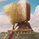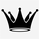5593 lượt xem
Alerts for famous Turtle Trading Rules. Based on work from tmr0 and ossettia. It can be used with Autoview.

If you have any advice on how to further improve the script, please let me know by PM or comment below. Thanks!

Please hit on "Like" if you find this script useful so that others can find it easier. Thanks!

*** The information and script provided here are no recommendation to buy or sell. They are just an idea for educational and discussion purposes. ***
Phát hành các Ghi chú: Alerts for famous Turtle Trading Rules. Based on work from tmr0 and ossettia. Compatible with Autoview.

Please "Like" if you find this script useful. Thanks!

Update v2: Label positioning and naming below (buy) and above (sell) bars added to better visualize entries and exits.

*** The information and script provided here are no recommendation to buy or sell. They are just an idea for educational and discussion purposes. ***
Phát hành các Ghi chú: Added background color: red = short / blue = long.

Please like if you find this script useful. Thanks!
Phát hành các Ghi chú:
• Updated to Pine Script v4
• Changed shape of label
• Changed label colors to better distinguish between fast and long signals

Please like if you find this script useful. Thanks!

## Bình luậnHere is my Autoview code for XBTUSD on BitMEX for the ones interested. Please adapt accordingly to your needs.

a=enter-fast-L d=1
a=bitmex-testnet e=bitmex-testnet s=XBTUSD b=long l=5 q=100 t=limit ro=0

a=enter-slow-L d=1
a=bitmex-testnet e=bitmex-testnet s=XBTUSD b=long l=5 q=100 t=limit ro=0

a=exit-fast-S d=1
a=bitmex-testnet e=bitmex-testnet s=XBTUSD b=long l=5 q=100 t=limit ro=1

a=exit-slow-S d=1
a=bitmex-testnet e=bitmex-testnet s=XBTUSD b=long l=5 q=100 t=limit ro=1

a=exit-fast-L d=1
a=bitmex-testnet e=bitmex-testnet s=XBTUSD b=short l=5 q=100 t=limit ro=1

a=exit-slow-L d=1
a=bitmex-testnet e=bitmex-testnet s=XBTUSD b=short l=5 q=100 t=limit ro=1

a=enter-fast-S d=1
a=bitmex-testnet e=bitmex-testnet s=XBTUSD b=short l=5 q=100 t=limit ro=0

a=enter-slow-S d=1
a=bitmex-testnet e=bitmex-testnet s=XBTUSD b=short l=5 q=100 t=limit ro=0
Phản hồiHello there
I have 2 questions:

1. What does these lines means in the script...
prevL1 = 0
prevL1 := exitL1_filt ? 1 : enterL1_filt ? -1 : prevL1
enterL1_final = enterL1_filt and prevL1 == 1
exitL1_final = exitL1_filt and prevL1 == -1
What does do " := " in Pine script. I'm newbie in Pine and this operator isn't working in Pine v.4 apparently. Am I right?

2.Does this script use N (Same ATR) for pyramiding and adding more units as explained in the original idea?

Thanks a lot for your time
Phản hồiMomoJumbo
@MomoJumbo, Hi MomoJumbo. Here are my answers:

1. There are some drawing issues when you convert from a strategy to a study with alerts. So you need to handle these issues with this code. Check out e.g. https://www.youtube.com/watch?v=KcBce_06OP0

2. No, there is no pyramiding used. Basically, the script above is just a conversion of the strategy into a study with alerts so you can use it with e.g. ProfitView.

Hope that helped.
Phản hồifiki
@fiki,

Thanks a lot
Can I ask some more help?

1. How can we have plotted the price (for example price of "enterL1_final") Instead of text (for example "OFL") beside the shape on chart?

2. Is there any way to plot shape aside the bar at the exact price Instead of below or above the bar?

3. I wanna add pyramiding & stop to script but couldn't say to the Compiler to get last entry price or last pyramiding price.
Is there any chance to get an specified price and use it for next calculations?

Thank you so much for your time.
Wish you luck
Phản hồihi, thanks for this, do you have the strategy for this in pine V4 ?
Phản hồiPrincecrypto
@Princecrypto, Hi Princecrypto. The strategy can be found in the article from tmr0 which you can find here:

The job I did is to translate the strategy into study so you can use automated alerts, e.g. with ProvitView.
Phản hồi